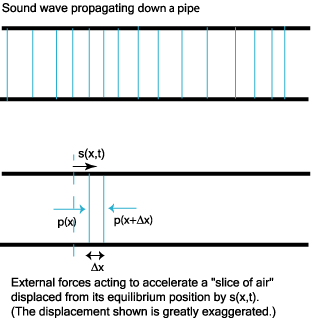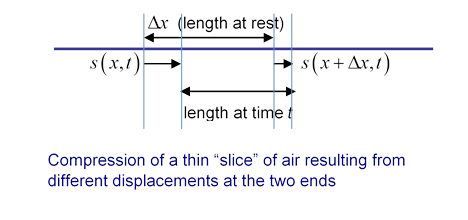# Sound Waves

Michael Fowler, University of Virginia

### “One-Dimensional” Sound Waves

We’ll begin by considering sound traveling down a hollow pipe, to avoid unnecessary mathematical complications. Sound is a longitudinal wave$—$as the wave passes through, the air moves backwards and forwards in the pipe, this oscillatory movement is in the same direction the wave is traveling.

To visualize what’s happening, imagine mentally dividing the air in the pipe, which is at rest if there is no sound, into a stack of thin slices.  Think about one of these slices. In equilibrium, it feels equal and opposite pressure from the gas on its two sides.  (This is analogous to the little bit of string at rest feeling equal and opposite tension on its two sides, but of course the gas pressure is inward).  As the sound wave goes through, the pressure wave generates slight differences in pressure on the two sides of our thin slice of air, and this imbalance of forces causes the slice to accelerate.

To analyze this quantitatively$—$to apply $F → =m a →$ to the thin slice of air$—$we must begin by defining displacement, the quantity corresponding to the string’s transverse movement $y( x,t ).$ We shall use $s( x,t )$ to denote the horizontal (along the pipe) displacement of the thin slice of air which rests at position $x$ when no sound is present.An animated version of this diagram is available here!

If the pipe has radius $a,$ and hence cross-sectional area $π a 2 ,$ a slice of air of thickness $Δx$ has volume $π a 2 Δx,$ so writing the density of air $ρ$ (1.29 kg/m3), the mass of the slice of air is $m=ρ V=ρπ a 2 Δ x.$  Clearly, its acceleration is $a= ∂ 2 s( x,t )/∂ t 2 ,$ so we already have the right-hand side of $F → =m a → .$  To find the left hand side$—$the force on the thin slice of air$—$we must find the pressure imbalance between the two sides.

### Relating Pressure Change to How the Displacement Varies

The pressure change as the sound wave moves down the tube is directly tied to the local compression or expansion of the gas.  It’s like a spring: as the gas is compressed into a smaller volume, its pressure rises, and as the gas expands the pressure drops.  And, exactly as for a spring, the changes in pressure and volume are linearly related.  The coefficient of proportionality is called the bulk modulus, usually written $B,$ and defined by the equation:

$Δp=−B ΔV V .$

Note the sign!  As the volume decreases, the pressure increases.  Since the ratio of volumes is dimensionless, the units for the bulk modulus are the same as for pressure: Pascals.  For air at standard temperature and pressure, the bulk modulus $B= 10 5$ Pa.

Now, we are tracking the motion of the gas as the sound wave passes through by following the parameter $s( x,t ),$ the displacement along the tube at time $t$ of gas having equilibrium position $x.$ Obviously, if $s( x,t )$ does not depend on $x,$ all the gas is shifted by the same amount, and no compression or expansion has taken place.  Local change in volume only happens if there is local variation in $s( x,t ).$

To make this quantitative, consider a slice of gas having thickness $Δ x$ (when at rest):  if, at some instant when the sound wave is passing through, the right-hand end is displaced by $s( x+Δx,t ),$ and the left-hand end by a greater amount $s( x,t ),$ say,the thickness of the slice has evidently been changed from $Δ x$ to

$Δx−( s( x,t )−s( x+Δx,t ) ).$

Since the volume of air in the slice is directly proportional to its thickness, the sound wave has at this instant changed the volume of the air initially in the segment $Δx$ near the point $x$ by a fraction

$ΔV V = s( x+Δx,t )−s( x,t ) Δx = ∂s( x,t ) ∂x ,$

the differential being exact in the limit of a thin slice.

Therefore, the local extra pressure is directly proportional to minus the gradient of $s( x,t ):$

$Δp=−B ΔV V =−B ∂s(x,t) ∂x .$.

### From F = ma  to the Wave Equation

Having found how the local pressure variation relates to $s( x,t ),$ we’re ready to derive the wave equation from $F=ma$ for a thin slice of gas.  Recall that for such a slice $m=ρ V=ρπ a 2 Δ x,$, and of course $a= ∂ 2 s( x,t )/∂ t 2 .$

The net force $F$ on the slice is the difference between the pressure at $x$ and that at $x+Δx:$

$F=p(x,t)π a 2 −p(x+Δx,t)π a 2 = −π a 2 B ∂s(x,t) ∂x +π a 2 B ∂s(x+Δx,t) ∂x =π a 2 BΔx ∂ 2 s(x,t) ∂ x 2$.

Putting this into $F=ma:$

This is exactly the wave equation we found for the string, with now the longitudinal displacement s replacing the transverse displacement $y,$ and the bulk modulus playing the role of the string tension, both being measures of stored potential energy arising from local variations in displacement.  The densities, of course, play the same role in the two cases, measuring how much kinetic energy is stored for given local displacement velocities.

### Boundary Conditions for Sound Waves in Pipes

Since the new wave equation is identical in form to that for waves on a string, our discussion of traveling waves, standing waves, etc., for a string can be carried over with the appropriate changes of notation and applied here.

For example, a standing wave in a pipe has the form $s( x,t )=Asinkxsinωt,$ this would be for a pipe closed at $x=0,$ so that the air doesn’t move at $x=0.$

The boundary condition for a closed end of a pipe is:

What about an open end?  In that case, the air is free to move$—$the boundary condition won’t be $s( x,t )=0.$ However, the pressure is not free to vary: it’s atmospheric pressure, the pipe being open to the atmosphere.  So at an open end $Δ p=0.$  Remembering that  $Δ​p=−B∂s( x,t )/∂x,$ the boundary condition is:

### Harmonic Standing Waves in Pipes

Consider now a standing harmonic wave in a pipe of length $L,$ closed at $x=0$ but open at $x=L.$

From the $x=0$ boundary condition, the wave must have the form  $s( x,t )=Asinkxsinωt.$

The $x=L$ open end boundary condition requires that the slope $∂s( L,t )/∂x=0.$

That is, $coskL=0.$

Exercise: Prove that the longest wavelength standing wave possible in the pipe has wavelength $4L,$ and sketch the wave.

Exercise: what is the next longest wavelength of a possible standing wave in the pipe?  Draw a picture.

### Traveling Waves: Power and Intensity

Another solution to the wave equation is

$s( x,t )=Asin( kx−ωt )$

where $ω=vk,$ just as for string.  This is a wave traveling down the pipe.  It could be generated by an oscillating plate at the closed end:  in other words, a speaker, just like the animation.

How much power is this speaker putting out?  It’s moving and pushing against the pressure:

Power = P = rate of working = force x velocity = pressure x area x velocity

How fast is it moving?  At time $t,$ the plate is at

$s( x=0,t )=−Asinωt,$

so it is moving at velocity

$v plate ( t )= ∂s( x=0,t ) ∂t =−Aωcosωt.$

The pressure at the plate is $Δp$ where

$Δp=−B ∂s(x,t) ∂x =−B ∂ ∂x Asin( kx−ωt )=−ABkcosωt$

at $x=0.$

Therefore, the rate of working at time $t,$ the power $P( t )=$ velocity x force:

$P( t )= v plate ( t )Δpπ a 2 = A 2 Bπ a 2 ωk cos 2 ωt.$

The standard definition of power for any kind of wave generator is the average power over a complete cycle.

Since the average value of $cos 2 x ¯ = 1 2 ,$

.

Using $B= v 2 ρ$ and $ω=vk,$, this can be written

$P= 1 2 A 2 π a 2 ω 2 ρv.$

This also tells us how much energy there is in the wave as it travels:

$1 2 A 2 π a 2 ω 2 ρ$ per meter.

The intensity of the wave is average power per square meter of cross sectional area, so here

and $I$ is measured in watts per square meter.

The factor $v,$ the velocity, in the above expression comes about because in one second, the energy delivered by a steady sound wave to one square meter of area perpendicular to the direction of the wave’s motion is the energy in $v$ cubic meters of wave: taking the speed of sound to be 330 meters per second, 330 cubic meters of sound energy will plough into one square meter each second.